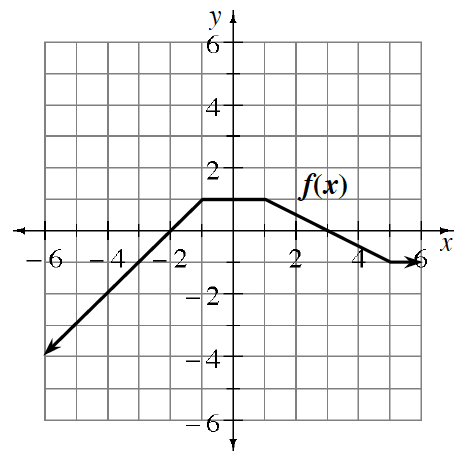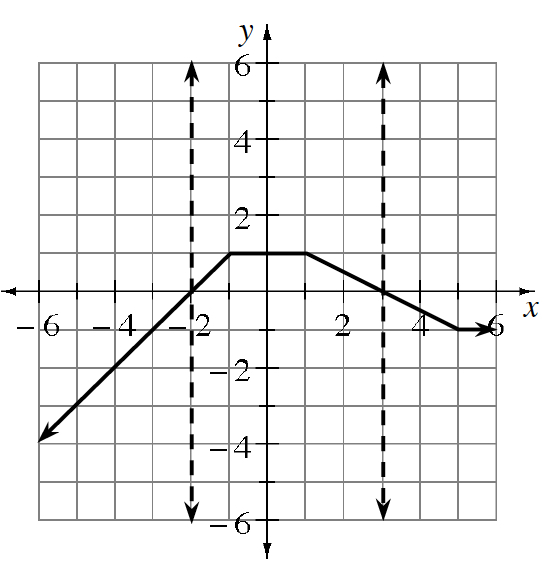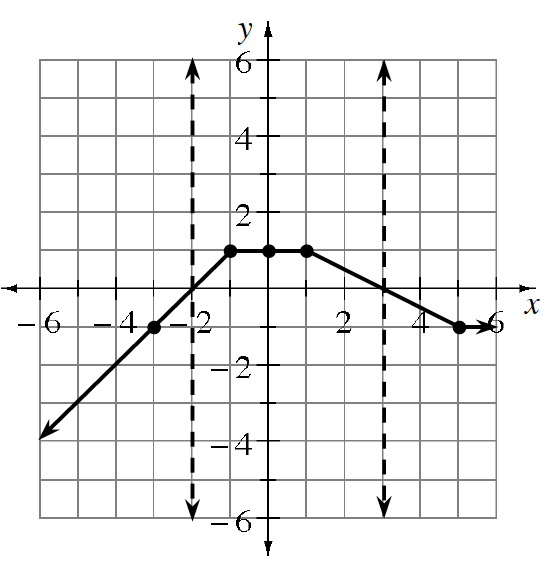### Home > PC3 > Chapter 5 > Lesson 5.2.2 > Problem5-74

5-74.

Using the graph of $y=f(x)$ below, sketch a graph of each of the following transformations.1. $y=−2f(x)$

1. Reflect vertically (across the $x$-axis).
2. Stretch vertically by a factor of $2$.

1. $y=f(−x)+1$

1. Reflect horizontally (across the $y$-axis).
2. Shift up $1$ unit.

1. $y=\frac{1}{f(x)}$

Sketch the vertical asymptotes. Added to the graph, 2 vertical dashed lines at, x = negative 2, & at, x = 3.

Since $\frac{1}{1}=1$ and $\frac{1}{-1}=-1$, mark the points that "stay in place" on the graph. The following Points highlighted: (negative 3, comma negative 1), (negative 1, comma 1), (0, comma 1), (1, comma 1), & (5, comma negative 1).

Where the original function is positive, the reciprocal function will also be positive.

Where the original function is negative, the reciprocal function will also be negative.